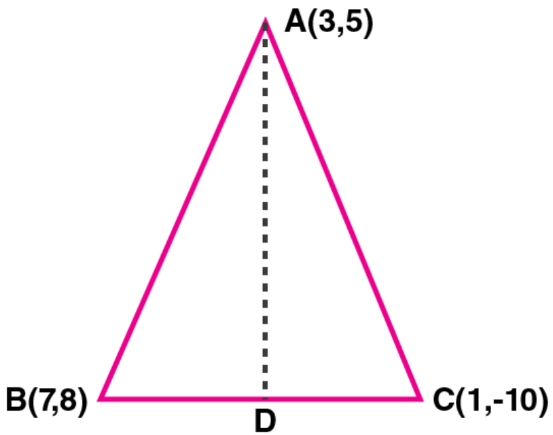Newbie

# In traingle ABC, A (3, 5), B (7, 8) and C (1, – 10). Find the equation of the median through A.

• 0

ICSE Board Question Based on Equation of a Straight Line Chapter of M.L Aggarwal for class10
Here a triangle ABC is given with their coordinates
You have to find the equation of the median through A.
This is the Question Number 23, Exercise 12.1 of M.L Aggarwal.

Share

1.Given,

∆ABC and their vertices A (3, 5), B (7, 8) and C (1, – 10).

So, D is mid-point of BC

Hence, the co-ordinates of D is ([7 + 1]/2, [8 – 10]/2) = (4, -1)

Now,

Slope of AD, m = y2 – y1/ x2 – x1

m = (5 + 1)/ (3 – 4) = 6/-1 = -6

Thus, the equation of AD is given by

y – y1 = m (x – x1)

y + 1 = -6 (x – 4)

y + 1 = -6x + 24

⇒ 6x + y – 23 = 0

• 0